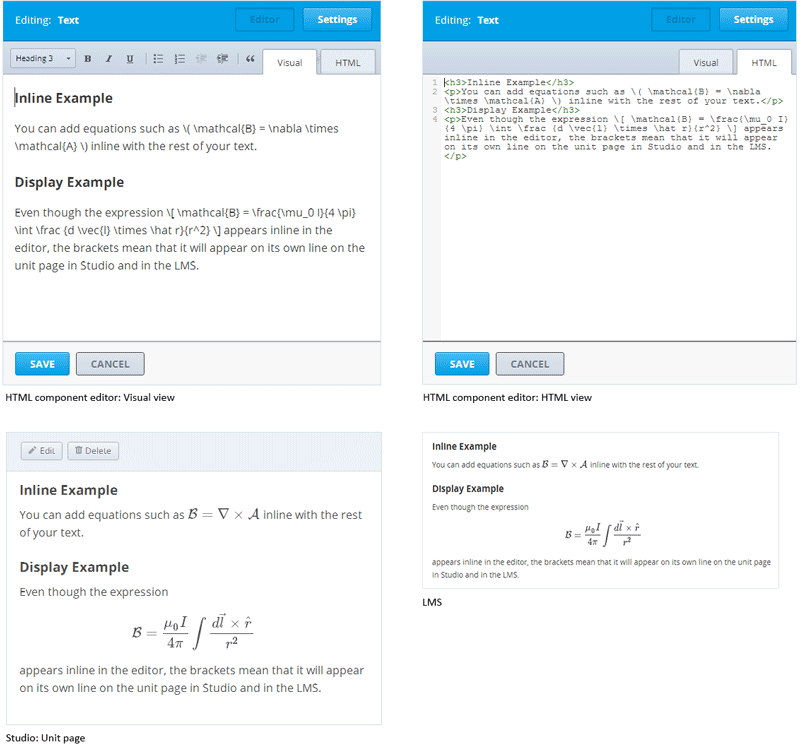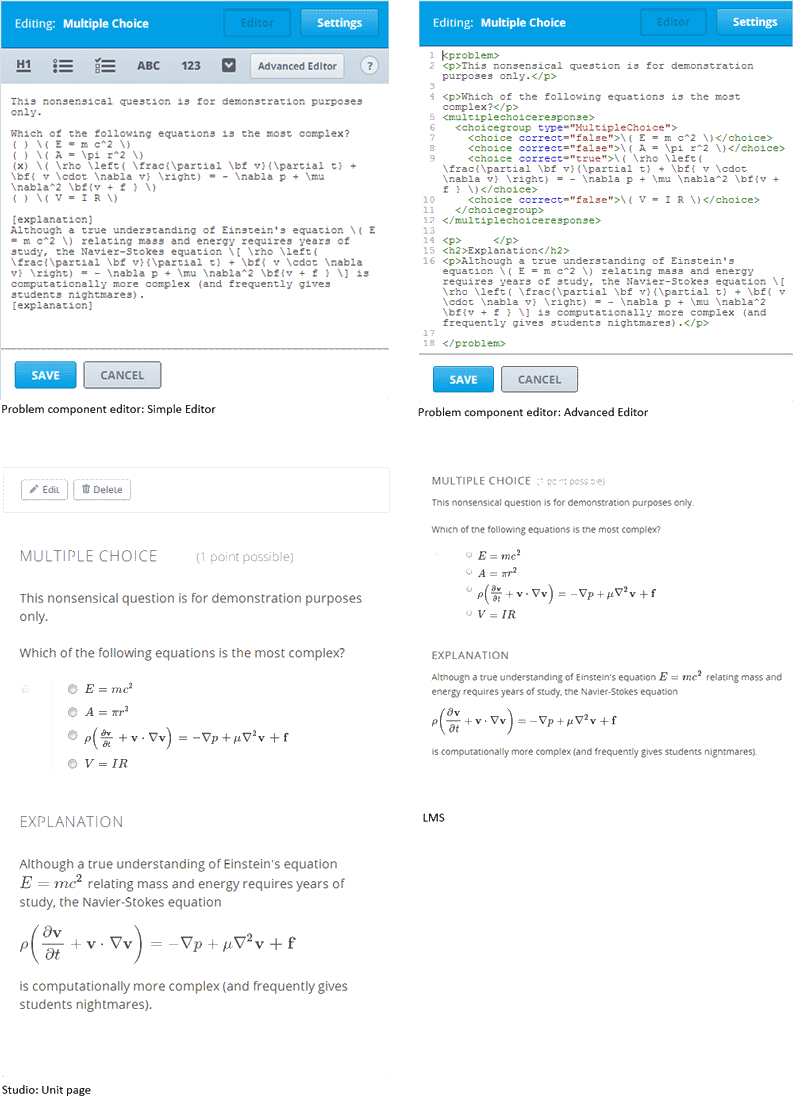# 10.47. Using MathJax for Mathematics¶

To produce clear and professional-looking symbols and equations in web browser, edX uses MathJax. MathJax automatically formats the mathematical symbols and equations that you enter in Text and problem components using LaTeX notation into beautiful math.

This topic provides some pointers to get you started. Many resources for learning and using MathJax are available online, including the official MathJax Documentation . A tutorial is available on the Mathematics meta stack exchange. Additional documentation, with a testing tool, is available on the Tree of Math site.

A MathJax equation can appear with other text in the paragraph (inline format) or on its own dedicated line (display format).

For inline equations, you can do either of the following.

• Surround your MathJax expression with backslash and parentheses characters.

$$equation$$

• Surround your MathJax expression with [mathjaxinline] tags. Note that these tags use brackets ([ ]).

[mathjaxinline] equation [/mathjaxinline]

For display equations, you can do either of the following.

• Surround your MathJax expression with backslash and bracket characters.

$equation$

• Surround your MathJax expression with [mathjax] tags. Note that these tags use brackets([ ])

[mathjax] equation [/mathjax]

## 10.47.1. Adding MathJax to Text Components¶

In the Text component editor, you can use MathJax in both visual view and HTML view.## 10.47.2. Adding MathJax to Problem Components¶

In the problem component editor, you can use MathJax in either the simple editor or advanced editor.

In the example that follows, note that the Einstein equation in the explanation is enclosed in backslashes and parentheses, so it appears inline with the text. The Navier-Stokes equation is enclosed in backslashes and brackets, so it appears on its own line (display).